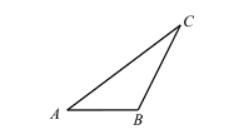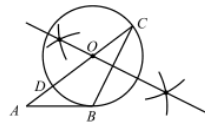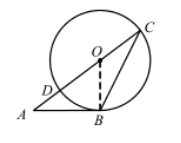(1) 求作 $\odot O$, 使得圆心 $O$ 在边 $A C$ 上, 且 $\odot O$ 经过点 $B 、 C$ (要求: 尺规作图, 不写作法, 保留作图癏迹);
(2) 在(1)的条件下, 设 $A C$ 与 $\odot O$ 的另一个交点为 $D$, 且 $A C=2 A B=4 A D$.(1)如图(2)\begin{aligned} & \therefore C D=A C-A D=8 k-2 k=6 k, \\ & \therefore O C=O B=O D=\frac{1}{2} \times 6 k=3 k, \\ & A O=A D+O D=2 k+3 k=5 k \quad \ldots \end{aligned}

$$\therefore O B^2+A B^2=A O^2 \text {, }$$
$\therefore \angle A B O=90^{\circ}$, 即 $A B \perp B O$.
$\because$ 点 $B$ 在 $\odot O$ 上,
$\therefore A B$ 是 $\odot O$ 的切线.
①点击 收藏 此题， 扫码注册关注公众号接收信息推送（一月四份试卷,中1+高2+研1）
② 程序开发、服务器资源都需要大量的钱，如果你感觉本站好或者受到到帮助，欢迎赞助本站,赞助方式：微信/支付宝转账到 18155261033

 ①此题难易度如何 ②此题推荐度如何 确定